# Chapter 7, Section 7.7, Question 07 Consider the following system of equations. (a) Find a fundamental matrix for the g...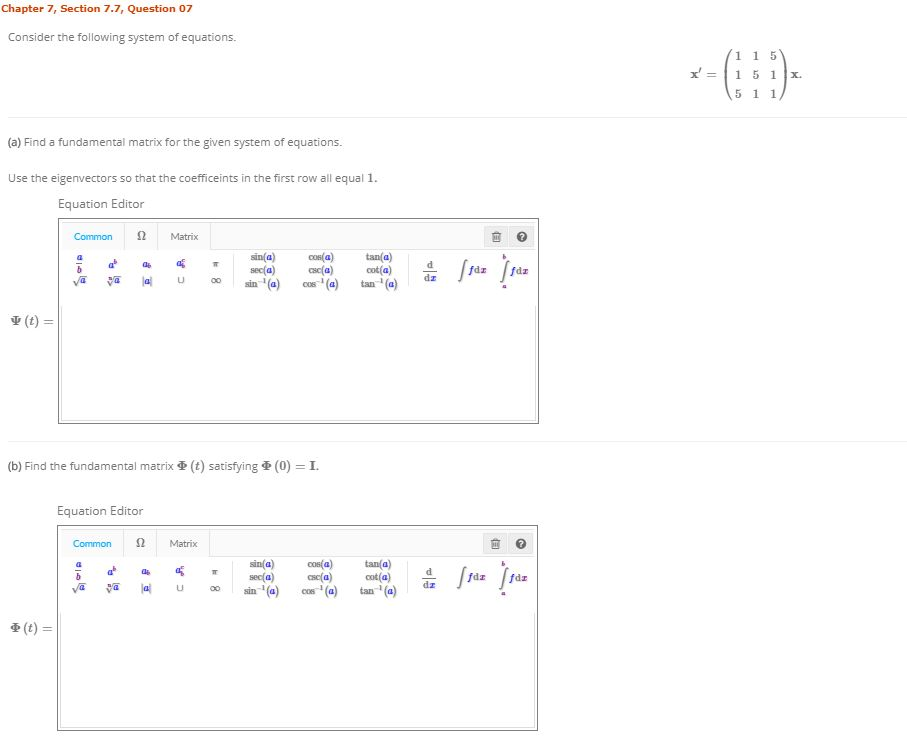Chapter 7, Section 7.7, Question 07 Consider the following system of equations. (a) Find a fundamental matrix for the given system of equations. Use the eigenvectors so that the coefficeints in the first row all equal 1 Equation Editor Ω Common Matrix Ψ (t) = (b) Find the fundamental matrix重(t) satisfying重(0) = 1. Equation Editor Ω Common Matrix tan a) sin(a) 0os(a) 重(t) =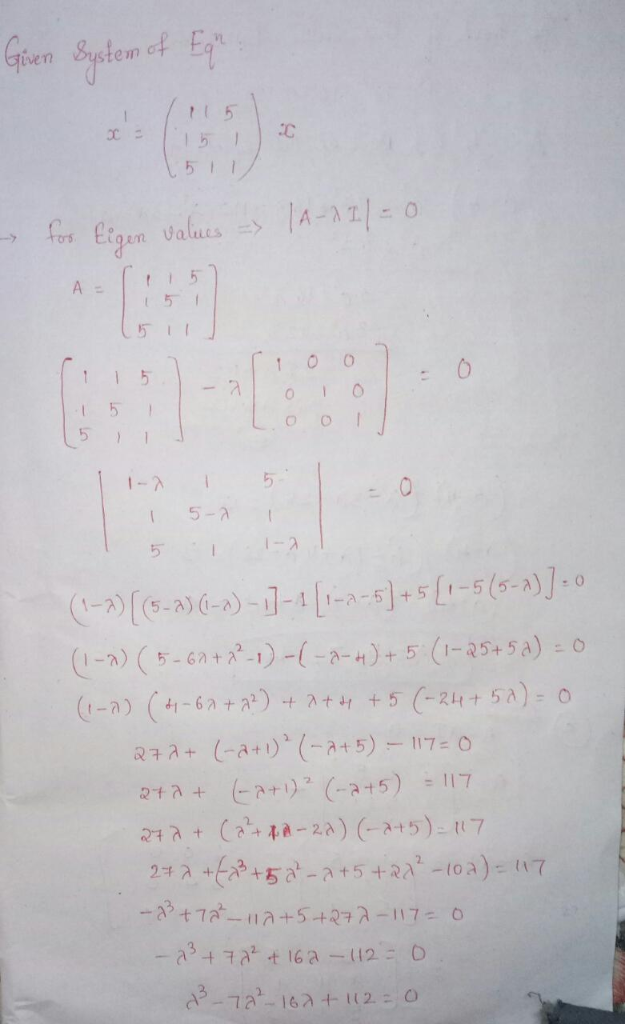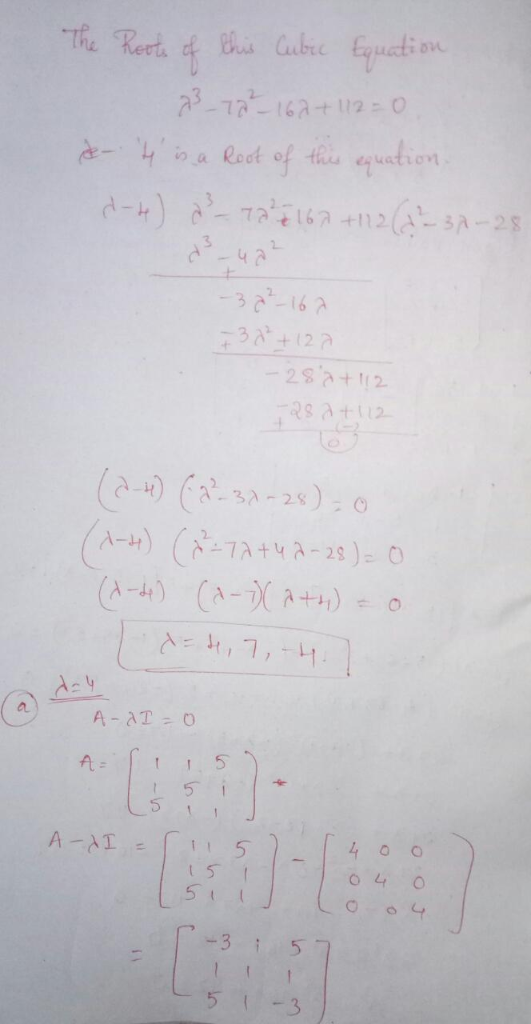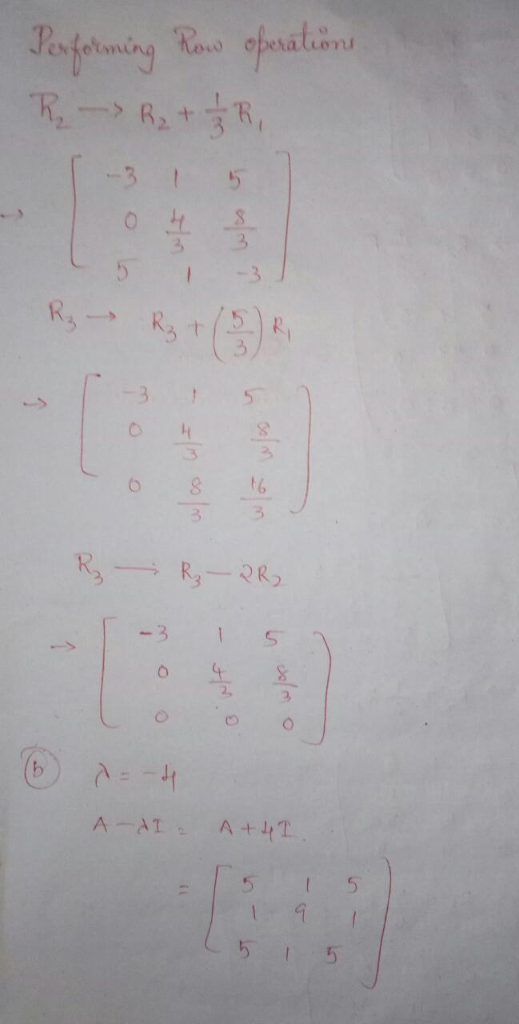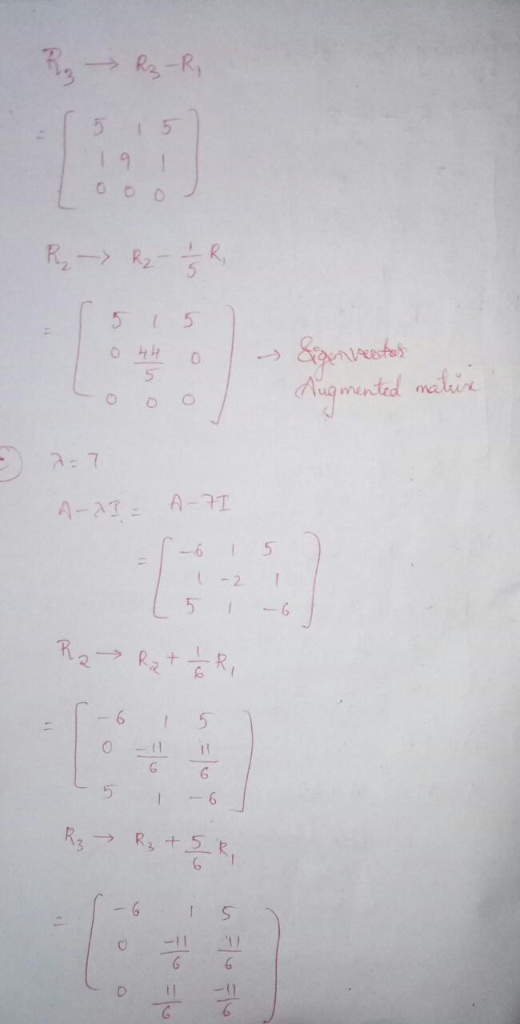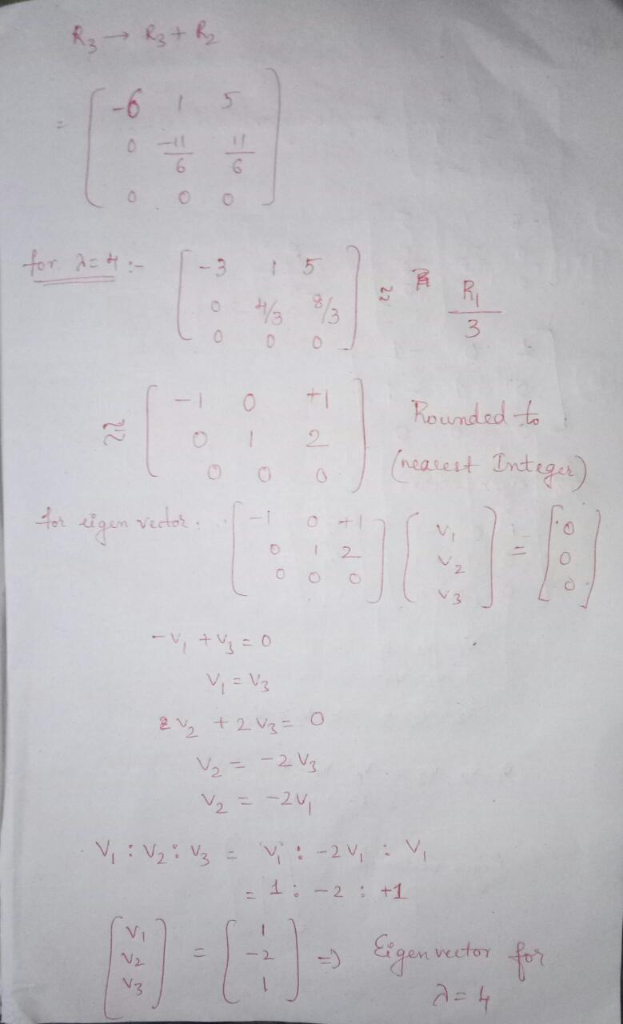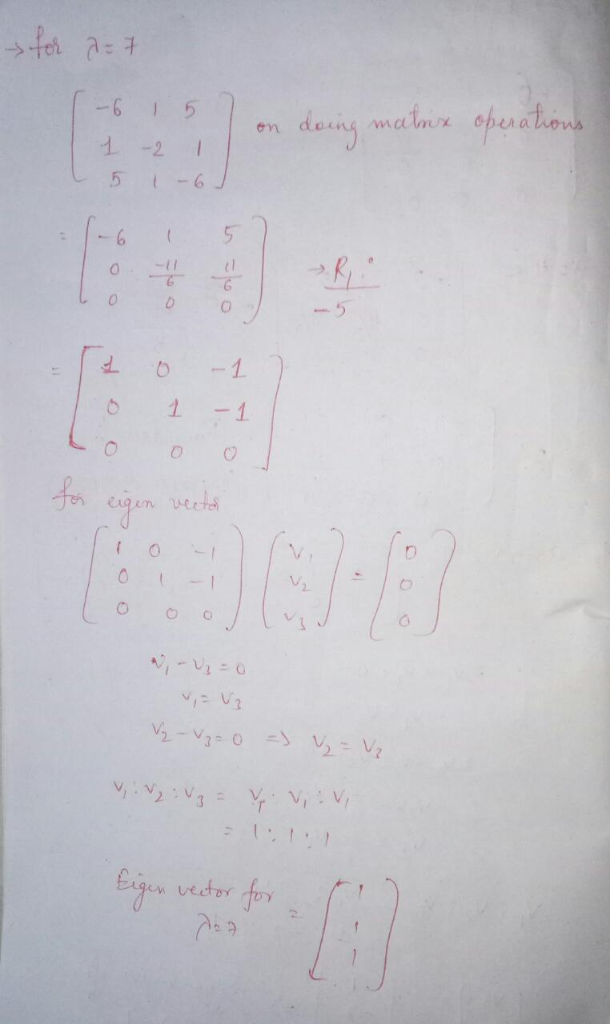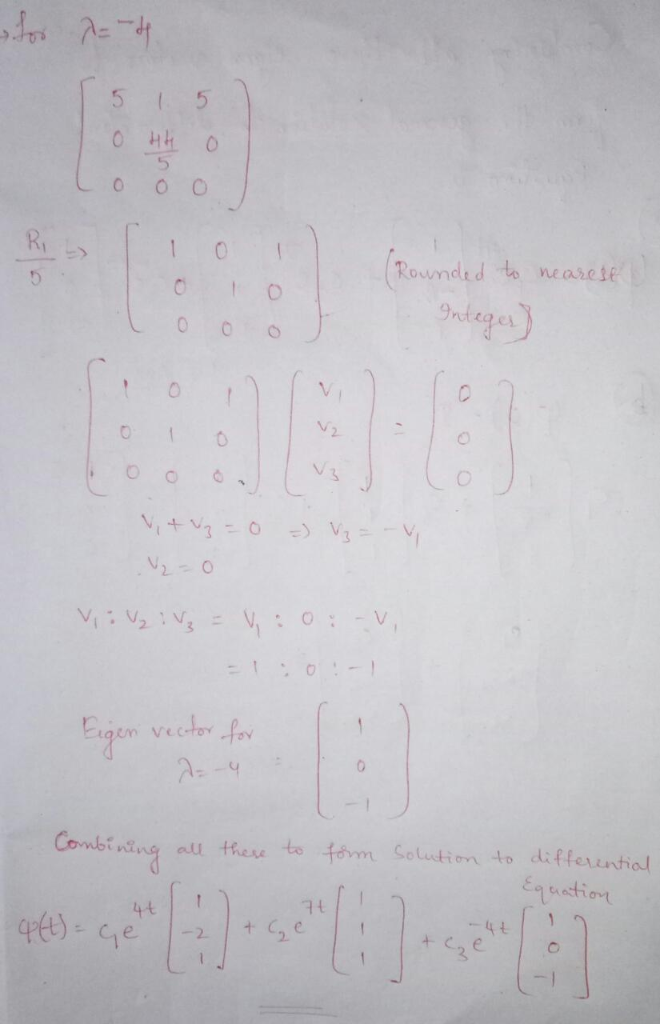i have solved only for a)

##### Add Answer of: Chapter 7, Section 7.7, Question 07 Consider the following system of equations. (a) Find a fundamental matrix for the g...
More Homework Help Questions Additional questions in this topic.